Finding Inverse

Chapter 1 Class 12 Relation and Functions
Concept wise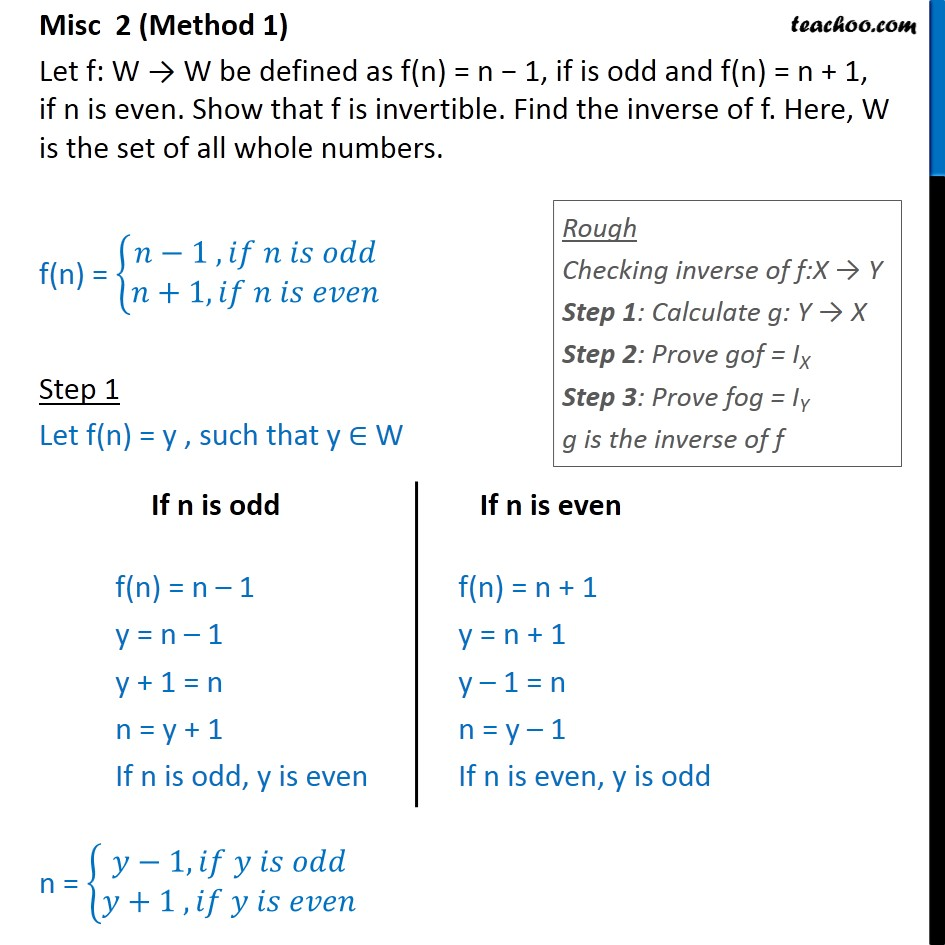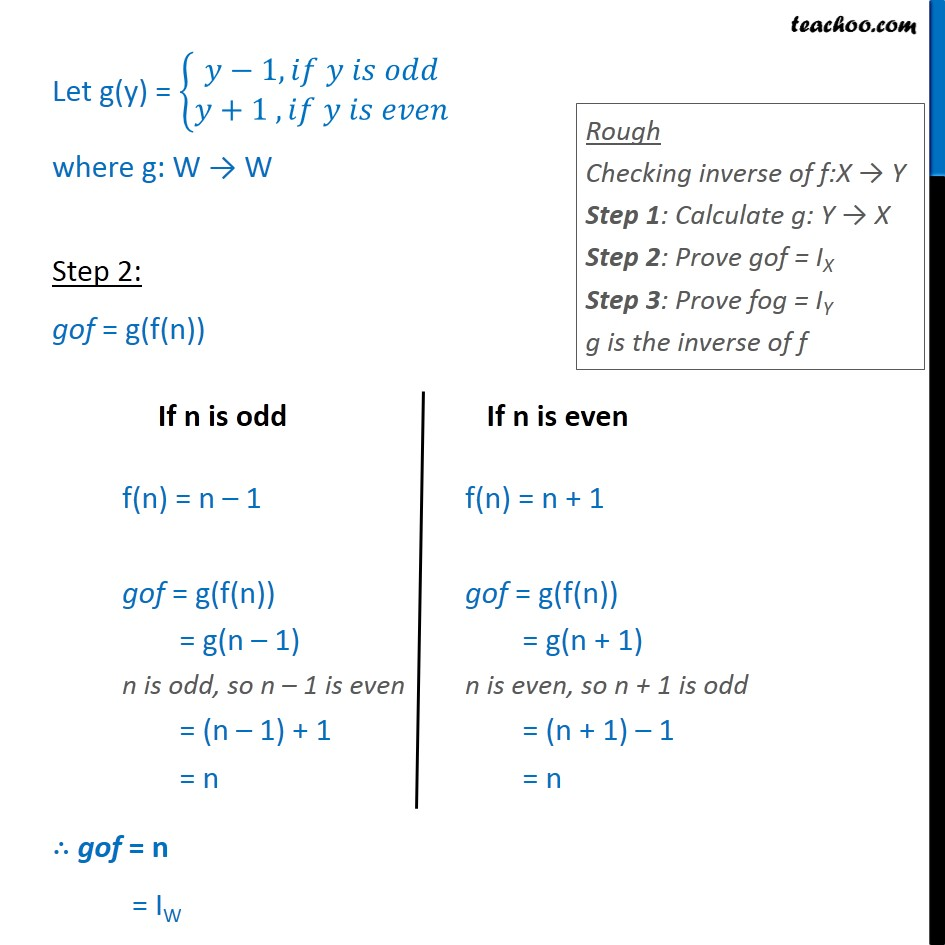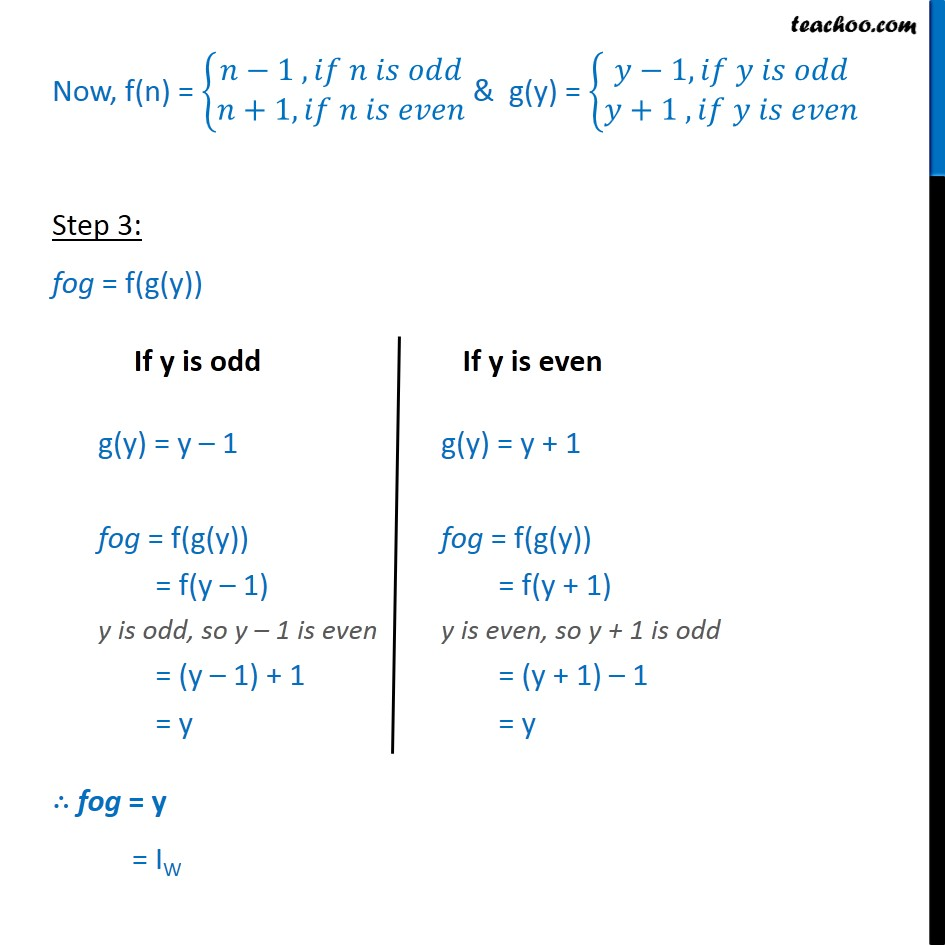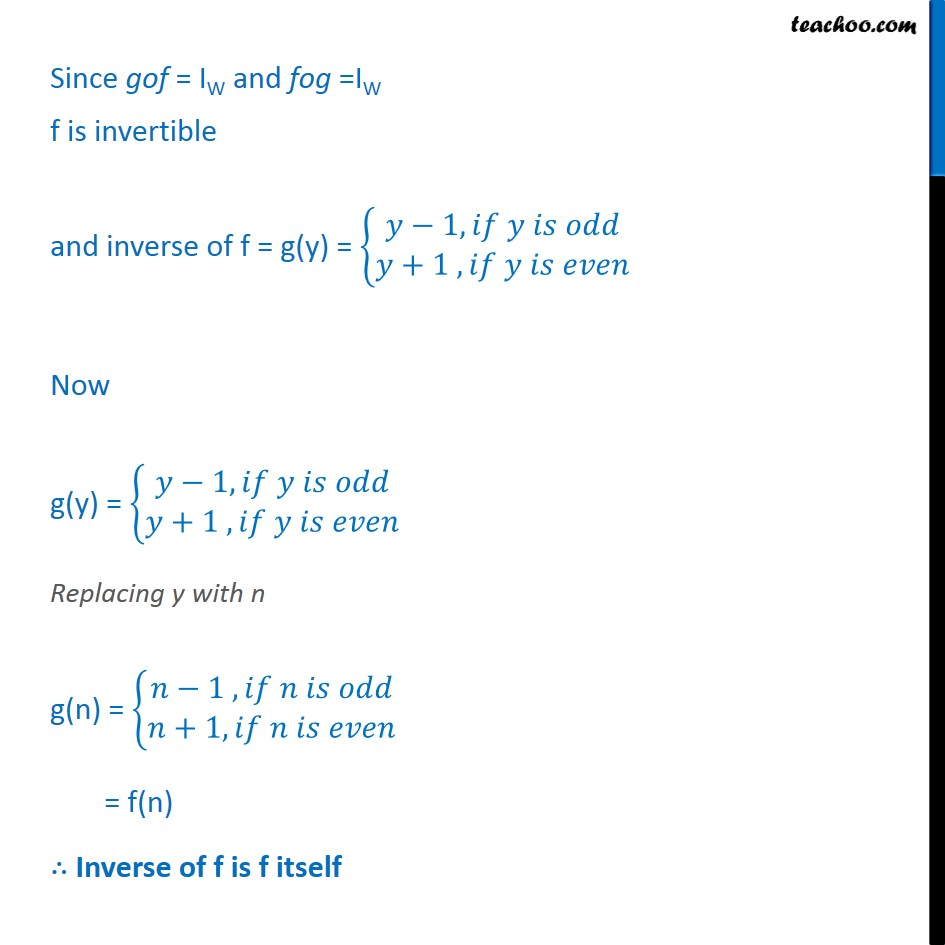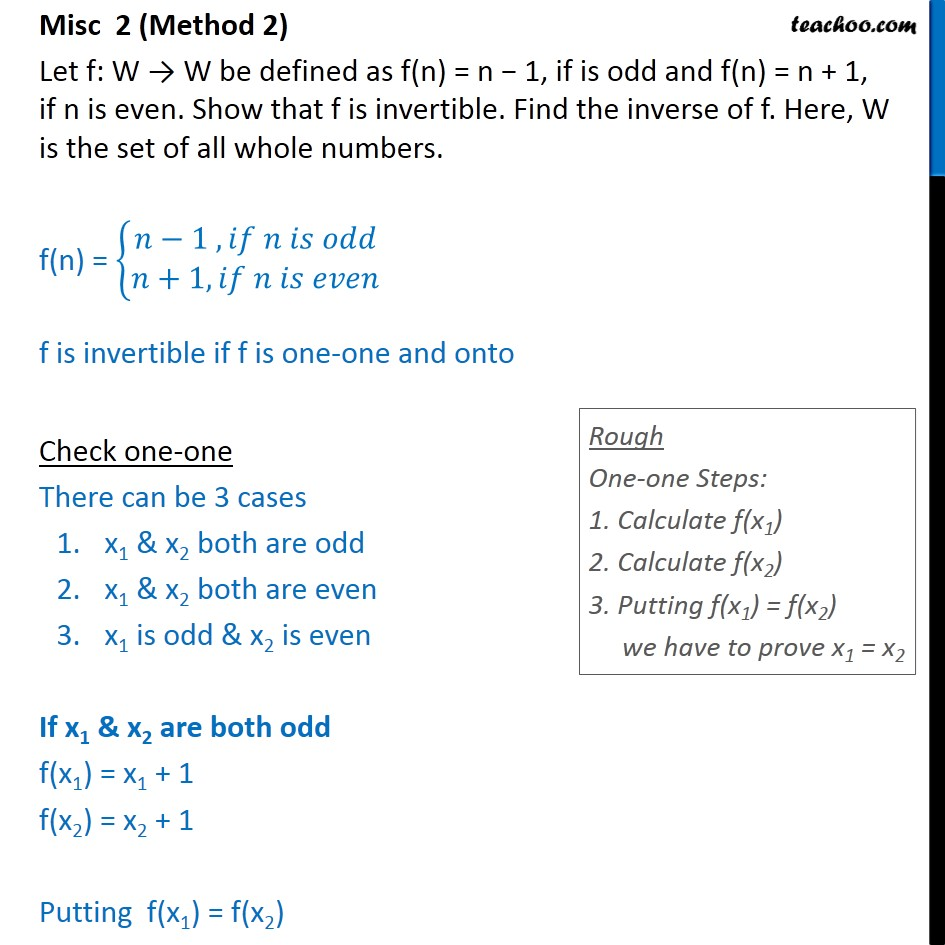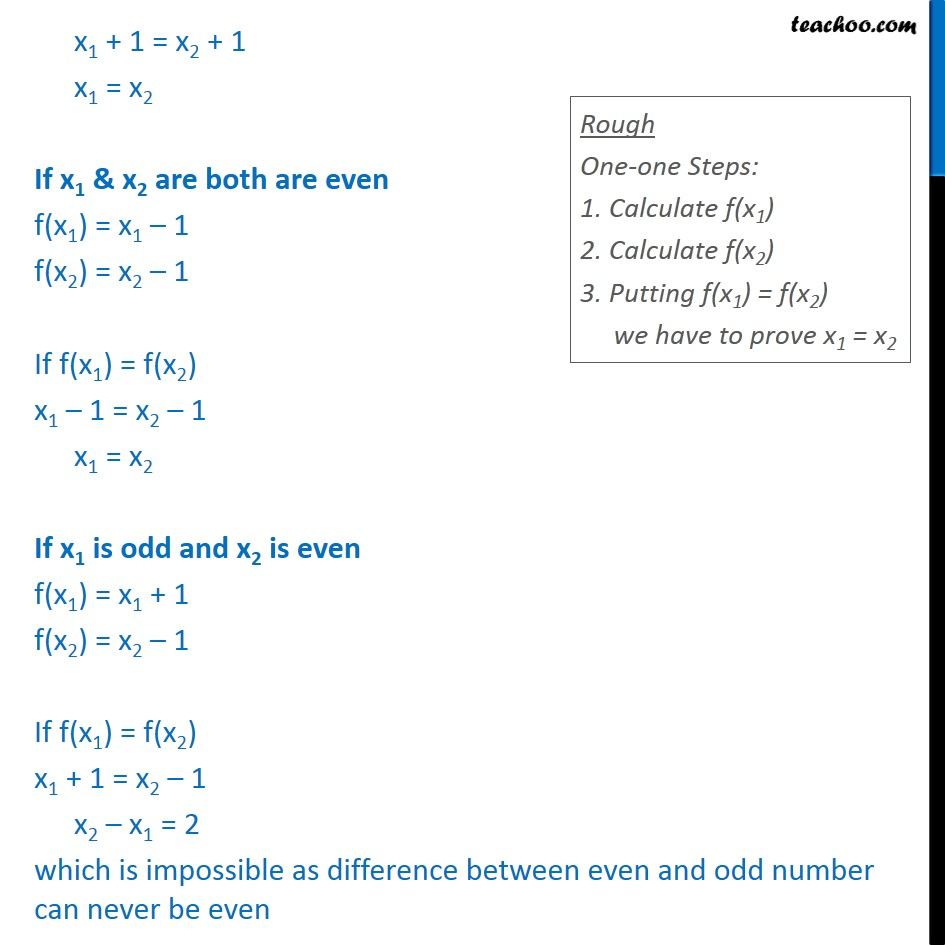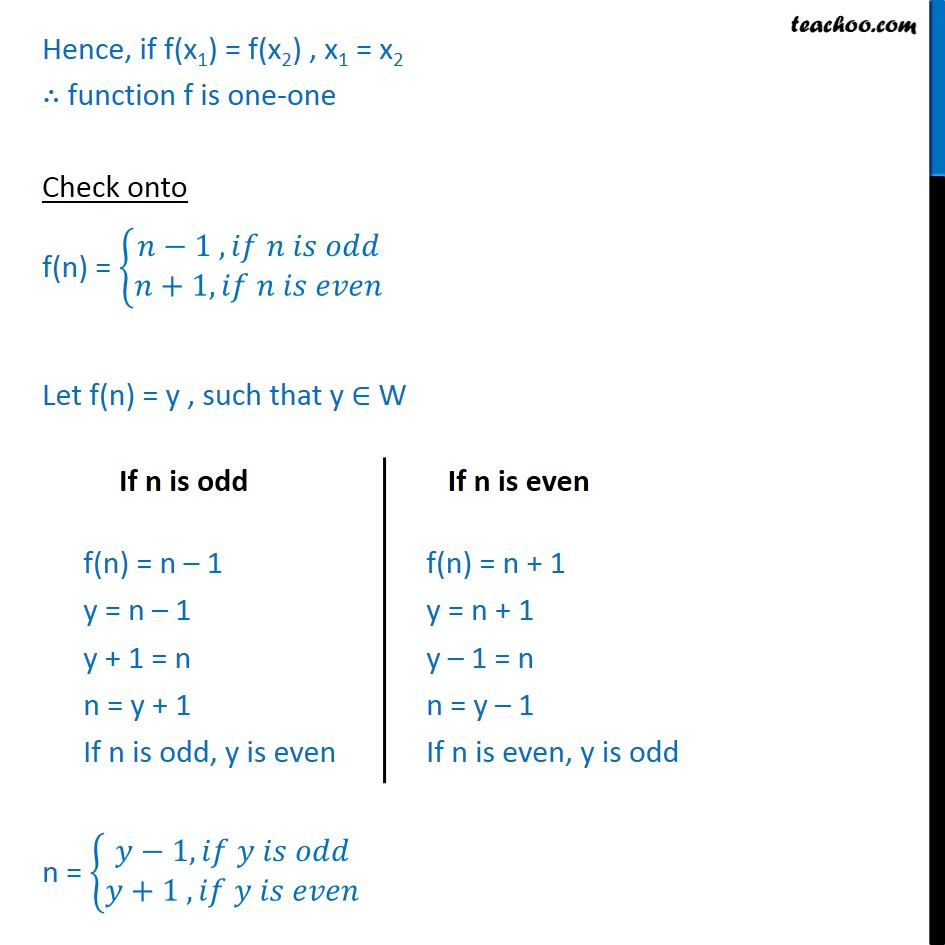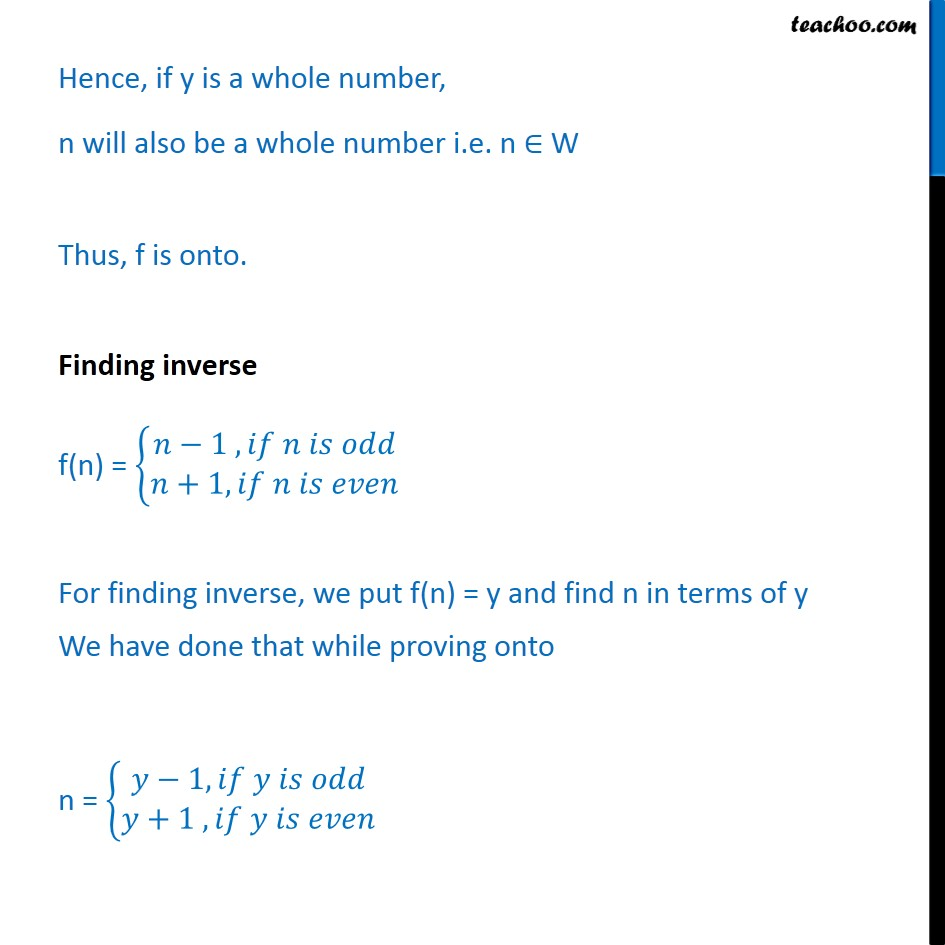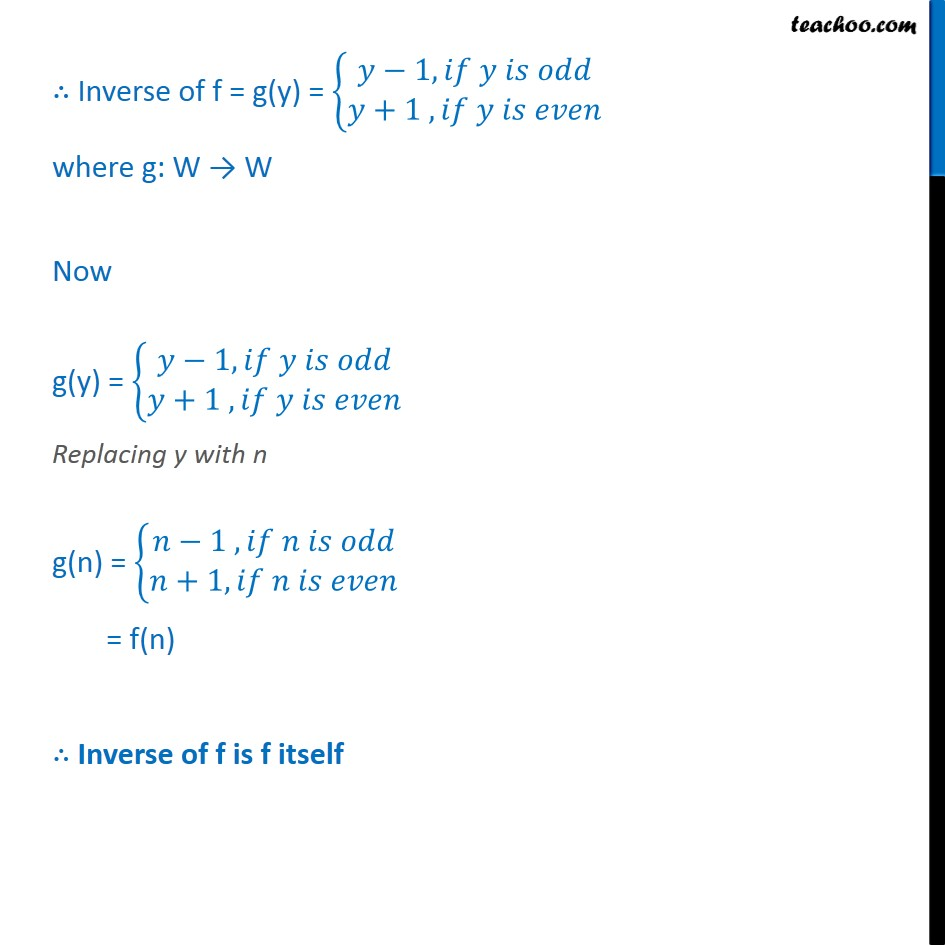Introducing your new favourite teacher - Teachoo Black, at only ₹83 per month

### Transcript

Misc 2 (Method 1) Let f: W → W be defined as f(n) = n − 1, if is odd and f(n) = n + 1, if n is even. Show that f is invertible. Find the inverse of f. Here, W is the set of all whole numbers. f(n) = 𝑛−1 , 𝑖𝑓 𝑛 𝑖𝑠 𝑜𝑑𝑑﷮𝑛+1, 𝑖𝑓 𝑛 𝑖𝑠 𝑒𝑣𝑒𝑛﷯﷯ Step 1 Let f(n) = y , such that y ∈ W n = 𝑦−1, 𝑖𝑓 𝑦 𝑖𝑠 𝑜𝑑𝑑﷮𝑦+1 , 𝑖𝑓 𝑦 𝑖𝑠 𝑒𝑣𝑒𝑛﷯﷯ Let g(y) = 𝑦−1, 𝑖𝑓 𝑦 𝑖𝑠 𝑜𝑑𝑑﷮𝑦+1 , 𝑖𝑓 𝑦 𝑖𝑠 𝑒𝑣𝑒𝑛﷯﷯ where g: W → W Step 2: gof = g(f(n)) ∴ gof = n = IW Now, f(n) = 𝑛−1 , 𝑖𝑓 𝑛 𝑖𝑠 𝑜𝑑𝑑﷮𝑛+1, 𝑖𝑓 𝑛 𝑖𝑠 𝑒𝑣𝑒𝑛﷯﷯ & g(y) = 𝑦−1, 𝑖𝑓 𝑦 𝑖𝑠 𝑜𝑑𝑑﷮𝑦+1 , 𝑖𝑓 𝑦 𝑖𝑠 𝑒𝑣𝑒𝑛﷯﷯ Step 3: fog = f(g(y)) ∴ fog = y = IW Since gof = IW and fog =IW f is invertible and inverse of f = g(y) = 𝑦−1, 𝑖𝑓 𝑦 𝑖𝑠 𝑜𝑑𝑑﷮𝑦+1 , 𝑖𝑓 𝑦 𝑖𝑠 𝑒𝑣𝑒𝑛﷯﷯ Now g(y) = 𝑦−1, 𝑖𝑓 𝑦 𝑖𝑠 𝑜𝑑𝑑﷮𝑦+1 , 𝑖𝑓 𝑦 𝑖𝑠 𝑒𝑣𝑒𝑛﷯﷯ Replacing y with n g(n) = 𝑛−1 , 𝑖𝑓 𝑛 𝑖𝑠 𝑜𝑑𝑑﷮𝑛+1, 𝑖𝑓 𝑛 𝑖𝑠 𝑒𝑣𝑒𝑛﷯﷯ = f(n) ∴ Inverse of f is f itself Misc 2 (Method 2) Let f: W → W be defined as f(n) = n − 1, if is odd and f(n) = n + 1, if n is even. Show that f is invertible. Find the inverse of f. Here, W is the set of all whole numbers. f(n) = 𝑛−1 , 𝑖𝑓 𝑛 𝑖𝑠 𝑜𝑑𝑑﷮𝑛+1, 𝑖𝑓 𝑛 𝑖𝑠 𝑒𝑣𝑒𝑛﷯﷯ f is invertible if f is one-one and onto Check one-one There can be 3 cases • x1 & x2 both are odd • x1 & x2 both are even • x1 is odd & x2 is even If x1 & x2 are both odd f(x1) = x1 + 1 f(x2) = x2 + 1 Putting f(x1) = f(x2) x1 + 1 = x2 + 1 x1 = x2 If x1 & x2 are both are even f(x1) = x1 – 1 f(x2) = x2 – 1 If f(x1) = f(x2) x1 – 1 = x2 – 1 x1 = x2 If x1 is odd and x2 is even f(x1) = x1 + 1 f(x2) = x2 – 1 If f(x1) = f(x2) x1 + 1 = x2 – 1 x2 – x1 = 2 which is impossible as difference between even and odd number can never be even Hence, if f(x1) = f(x2) , x1 = x2 ∴ function f is one-one Check onto f(n) = 𝑛−1 , 𝑖𝑓 𝑛 𝑖𝑠 𝑜𝑑𝑑﷮𝑛+1, 𝑖𝑓 𝑛 𝑖𝑠 𝑒𝑣𝑒𝑛﷯﷯ Let f(n) = y , such that y ∈ W n = 𝑦−1, 𝑖𝑓 𝑦 𝑖𝑠 𝑜𝑑𝑑﷮𝑦+1 , 𝑖𝑓 𝑦 𝑖𝑠 𝑒𝑣𝑒𝑛﷯﷯ Hence, if y is a whole number, n will also be a whole number i.e. n ∈ W Thus, f is onto. Finding inverse f(n) = 𝑛−1 , 𝑖𝑓 𝑛 𝑖𝑠 𝑜𝑑𝑑﷮𝑛+1, 𝑖𝑓 𝑛 𝑖𝑠 𝑒𝑣𝑒𝑛﷯﷯ For finding inverse, we put f(n) = y and find n in terms of y We have done that while proving onto n = 𝑦−1, 𝑖𝑓 𝑦 𝑖𝑠 𝑜𝑑𝑑﷮𝑦+1 , 𝑖𝑓 𝑦 𝑖𝑠 𝑒𝑣𝑒𝑛﷯﷯ ∴ Inverse of f = g(y) = 𝑦−1, 𝑖𝑓 𝑦 𝑖𝑠 𝑜𝑑𝑑﷮𝑦+1 , 𝑖𝑓 𝑦 𝑖𝑠 𝑒𝑣𝑒𝑛﷯﷯ where g: W → W Now g(y) = 𝑦−1, 𝑖𝑓 𝑦 𝑖𝑠 𝑜𝑑𝑑﷮𝑦+1 , 𝑖𝑓 𝑦 𝑖𝑠 𝑒𝑣𝑒𝑛﷯﷯ Replacing y with n g(n) = 𝑛−1 , 𝑖𝑓 𝑛 𝑖𝑠 𝑜𝑑𝑑﷮𝑛+1, 𝑖𝑓 𝑛 𝑖𝑠 𝑒𝑣𝑒𝑛﷯﷯ = f(n) ∴ Inverse of f is f itself